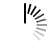# Reservoir Sampling

The Reservoir sampling is a randomized algorithm. In this algorithm, k items are chosen from a list with n different items.

We can solve it by creating an array as a reservoir of size k. Then randomly pick one element from the main list and placed that item in the reservoir list. When one item is selected once, it will not be selected for next time. But his approach is not effective, we can increase the complexity by this method.

In the reservoir list, copy first k items from the list, now one by one from the (k+1)th number in the list, let the current selected item is placed at index i. Find a random index from 0 to i and store it into j, if j is in the range of 0 to k, then swap reservoir[j] with list[i].

#### Input and Output

```Input:
The list of integers: {1, 2, 3, 4, 5, 6, 7, 8, 9, 10, 11, 12}, The value of k = 6
Output:
K-Selected items in the given array: 8 2 7 9 12 6```

#### Algorithm

`chooseKItems(array, n, k)`

Input: The array, number of elements in the array, number of elements to pick.

Output: Pick k elements randomly.

```Begin
define output array of size [k]
copy k first items from array to output

while i < n, do
j := randomly choose one value from 0 to i
if j < k, then
output[j] := array[i]
increase i by 1
done
display output array
End

```

#### Source Code (C++)

```#include <iostream>
#include <cstdlib>
#include <ctime>
using namespace std;

void display(int array[], int n) {
for (int i = 0; i < n; i++)
cout << array[i] << " ";
}

void chooseKItems(int array[], int n, int k) {        //it will choose k items from the array
int i;
int output[k];
for (i = 0; i < k; i++)
output[i] = array[i];

srand(time(NULL));        //use time function to get different seed value

while(i < n) {
int j = rand() % (i+1);         //random index from 0 to i

if (j < k)          //copy ith element to jth element in the output array
output[j] = array[i];
i++;
}

cout << "K-Selected items in the given array: ";
display(output, k);
}

int main() {
int array[] = {1, 2, 3, 4, 5, 6, 7, 8, 9, 10, 11, 12};
int n = 12;
int k = 6;
chooseKItems(array, n, k);
}```

#### Output

`K-Selected items in the given array: 8 2 7 9 12 6`